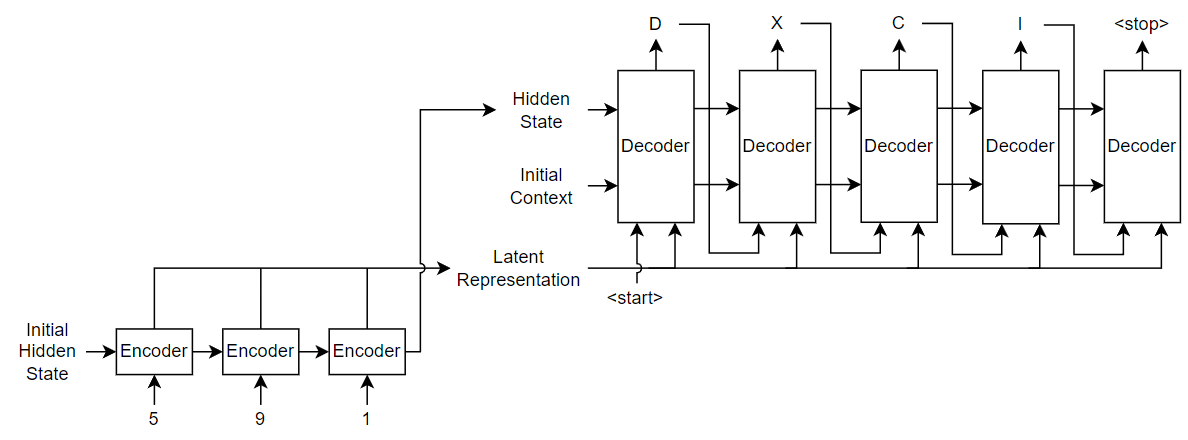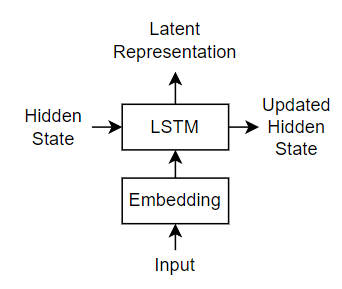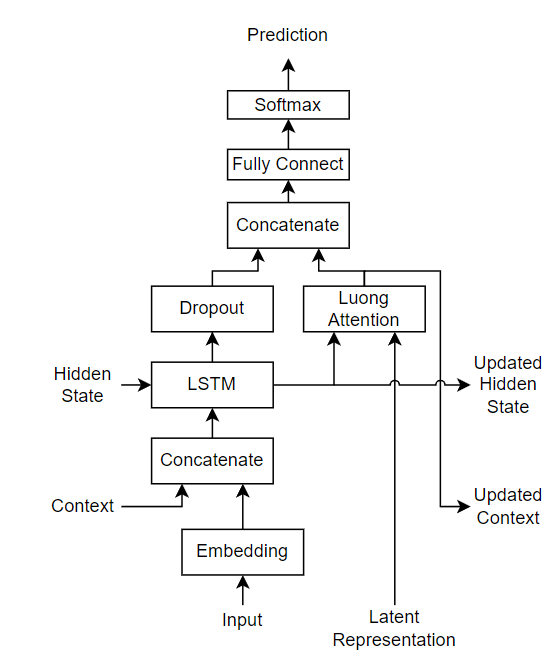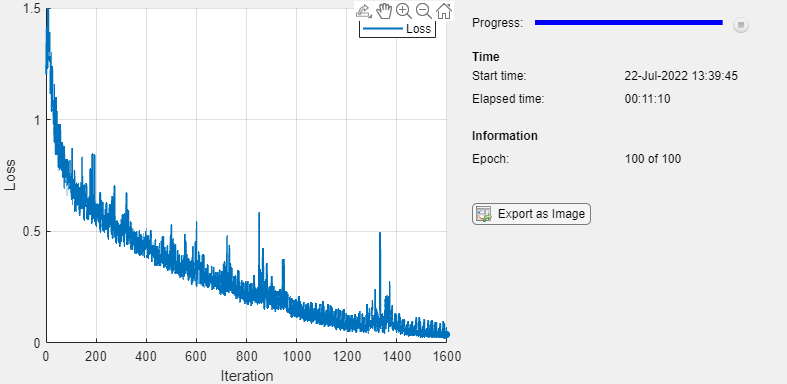Sequence-to-Sequence Translation Using Attention

This example shows how to convert decimal strings to Roman numerals using a recurrent sequence-to-sequence encoder-decoder model with attention.

Recurrent encoder-decoder models have proven successful at tasks like abstractive text summarization and neural machine translation. The model consists of an encoder which typically processes input data with a recurrent layer such as LSTM, and a decoder which maps the encoded input into the desired output, typically with a second recurrent layer. Models that incorporate attention mechanisms into the models allows the decoder to focus on parts of the encoded input while generating the translation.For the encoder model, this example uses a simple network consisting of an embedding followed by two LSTM operations. Embedding is a method of converting categorical tokens into numeric vectors.For the decoder model, this example uses a network very similar to the encoder that contains two LSTMs. However, an important difference is that the decoder contains an attention mechanism. The attention mechanism allows the decoder to attend to specific parts of the encoder output.filename = fullfile("romanNumerals.csv");

options = detectImportOptions(filename, ...
'TextType','string', ...
options.VariableNames = ["Source" "Target"];
options.VariableTypes = ["string" "string"];

Split the data into training and test partitions containing 50% of the data each.

idx = randperm(size(data,1),500);
dataTrain = data(idx,:);
dataTest = data;
dataTest(idx,:) = [];

View some of the decimal-Roman numeral pairs.

ans=8×2 table
Source     Target
______    ________

"492"     "CDXCII"
"911"     "CMXI"
"965"     "CMLXV"
"951"     "CMLI"
"160"     "CLX"
"662"     "DCLXII"
"102"     "CII"
"440"     "CDXL"

Preprocess Data

Preprocess the text data using the transformText function, listed at the end of the example. The transformText function preprocesses and tokenizes the input text for translation by splitting the text into characters and adding start and stop tokens. To translate text by splitting the text into words instead of characters, skip the first step.

startToken = "<start>";
stopToken = "<stop>";

strSource = dataTrain.Source;
documentsSource = transformText(strSource,startToken,stopToken);

Create a wordEncoding object that maps tokens to a numeric index and vice-versa using a vocabulary.

encSource = wordEncoding(documentsSource);

Using the word encoding, convert the source text data to numeric sequences.

Convert the target data to sequences using the same steps.

strTarget = dataTrain.Target;
documentsTarget = transformText(strTarget,startToken,stopToken);
encTarget = wordEncoding(documentsTarget);

Sort the sequences by length. Training with the sequences sorted by increasing sequence length results in batches with sequences of approximately the same sequence length and ensures smaller sequence batches are used to update the model before longer sequence batches.

sequenceLengths = cellfun(@(sequence) size(sequence,2),sequencesSource);
[~,idx] = sort(sequenceLengths);
sequencesSource = sequencesSource(idx);
sequencesTarget = sequencesTarget(idx);

Create arrayDatastore objects containing the source and target data and combine them using the combine function.

sequencesSourceDs = arrayDatastore(sequencesSource,'OutputType','same');
sequencesTargetDs = arrayDatastore(sequencesTarget,'OutputType','same');

sequencesDs = combine(sequencesSourceDs,sequencesTargetDs);

Initialize Model Parameters

Initialize the model parameters. for both the encoder and decoder, specify an embedding dimension of 128, two LSTM layers with 200 hidden units, and dropout layers with random dropout with probability 0.05.

embeddingDimension = 128;
numHiddenUnits = 200;
dropout = 0.05;

Initialize Encoder Model Parameters

Initialize the weights of the encoding embedding using the Gaussian using the initializeGaussian function which is attached to this example as a supporting file. Specify a mean of 0 and a standard deviation of 0.01. To learn more, see Gaussian Initialization.

inputSize = encSource.NumWords + 1;
sz = [embeddingDimension inputSize];
mu = 0;
sigma = 0.01;
parameters.encoder.emb.Weights = initializeGaussian(sz,mu,sigma);

Initialize the learnable parameters for the encoder LSTM operations:

• Initialize the input weights with the Glorot initializer using the initializeGlorot function which is attached to this example as a supporting file. To learn more, see Glorot Initialization.

• Initialize the recurrent weights with the orthogonal initializer using the initializeOrthogonal function which is attached to this example as a supporting file. To learn more, see Orthogonal Initialization.

• Initialize the bias with the unit forget gate initializer using the initializeUnitForgetGate function which is attached to this example as a supporting file. To learn more, see Unit Forget Gate Initialization.

Initialize the learnable parameters for the first encoder LSTM operation.

sz = [4*numHiddenUnits embeddingDimension];
numOut = 4*numHiddenUnits;
numIn = embeddingDimension;

parameters.encoder.lstm1.InputWeights = initializeGlorot(sz,numOut,numIn);
parameters.encoder.lstm1.RecurrentWeights = initializeOrthogonal([4*numHiddenUnits numHiddenUnits]);
parameters.encoder.lstm1.Bias = initializeUnitForgetGate(numHiddenUnits);

Initialize the learnable parameters for the second encoder LSTM operation.

sz = [4*numHiddenUnits numHiddenUnits];
numOut = 4*numHiddenUnits;
numIn = numHiddenUnits;

parameters.encoder.lstm2.InputWeights = initializeGlorot(sz,numOut,numIn);
parameters.encoder.lstm2.RecurrentWeights = initializeOrthogonal([4*numHiddenUnits numHiddenUnits]);
parameters.encoder.lstm2.Bias = initializeUnitForgetGate(numHiddenUnits);

Initialize Decoder Model Parameters

Initialize the weights of the encoding embedding using the Gaussian using the initializeGaussian function. Specify a mean of 0 and a standard deviation of 0.01.

outputSize = encTarget.NumWords + 1;
sz = [embeddingDimension outputSize];
mu = 0;
sigma = 0.01;
parameters.decoder.emb.Weights = initializeGaussian(sz,mu,sigma);

Initialize the weights of the attention mechanism using the Glorot initializer using the initializeGlorot function.

sz = [numHiddenUnits numHiddenUnits];
numOut = numHiddenUnits;
numIn = numHiddenUnits;
parameters.decoder.attn.Weights = initializeGlorot(sz,numOut,numIn);

Initialize the learnable parameters for the decoder LSTM operations:

• Initialize the input weights with the Glorot initializer using the initializeGlorot function.

• Initialize the recurrent weights with the orthogonal initializer using the initializeOrthogonal function.

• Initialize the bias with the unit forget gate initializer using the initializeUnitForgetGate function.

Initialize the learnable parameters for the first decoder LSTM operation.

sz = [4*numHiddenUnits embeddingDimension+numHiddenUnits];
numOut = 4*numHiddenUnits;
numIn = embeddingDimension + numHiddenUnits;

parameters.decoder.lstm1.InputWeights = initializeGlorot(sz,numOut,numIn);
parameters.decoder.lstm1.RecurrentWeights = initializeOrthogonal([4*numHiddenUnits numHiddenUnits]);
parameters.decoder.lstm1.Bias = initializeUnitForgetGate(numHiddenUnits);

Initialize the learnable parameters for the second decoder LSTM operation.

sz = [4*numHiddenUnits numHiddenUnits];
numOut = 4*numHiddenUnits;
numIn = numHiddenUnits;

parameters.decoder.lstm2.InputWeights = initializeGlorot(sz,numOut,numIn);
parameters.decoder.lstm2.RecurrentWeights = initializeOrthogonal([4*numHiddenUnits numHiddenUnits]);
parameters.decoder.lstm2.Bias = initializeUnitForgetGate(numHiddenUnits);

Initialize the learnable parameters for the decoder fully connected operation:

• Initialize the weights with the Glorot initializer.

• Initialize the bias with zeros using the initializeZeros function which is attached to this example as a supporting file. To learn more, see Zeros Initialization.

sz = [outputSize 2*numHiddenUnits];
numOut = outputSize;
numIn = 2*numHiddenUnits;

parameters.decoder.fc.Weights = initializeGlorot(sz,numOut,numIn);
parameters.decoder.fc.Bias = initializeZeros([outputSize 1]);

Define Model Functions

Create the functions modelEncoder and modelDecoder, listed at the end of the example, that compute the outputs of the encoder and decoder models, respectively.

The modelEncoder function, listed in the Encoder Model Function section of the example, takes the input data, the model parameters, the optional mask that is used to determine the correct outputs for training and returns the model outputs and the LSTM hidden state.

The modelDecoder function, listed in the Decoder Model Function section of the example, takes the input data, the model parameters, the context vector, the LSTM initial hidden state, the outputs of the encoder, and the dropout probability and outputs the decoder output, the updated context vector, the updated LSTM state, and the attention scores.

Create the function modelGradients, listed in the Model Gradients Function section of the example, that takes the encoder and decoder model parameters, a mini-batch of input data and the padding masks corresponding to the input data, and the dropout probability and returns the gradients of the loss with respect to the learnable parameters in the models and the corresponding loss.

Specify Training Options

Train with a mini-batch size of 32 for 75 epochs with a learning rate of 0.002.

miniBatchSize = 32;
numEpochs = 75;
learnRate = 0.002;

Train Model

Train the model using a custom training loop. Use minibatchqueue to process and manage mini-batches of images during training. For each mini-batch:

• Use the custom mini-batch preprocessing function preprocessMiniBatch (defined at the end of this example) to find the lengths of all sequence in the mini-batch and pad the sequences to the same length as the longest sequence, for the source and target sequences, respectively.

• Permute the second and third dimensions of the padded sequences.

• Return the mini-batch variables unformatted dlarray objects with underlying data type single. All other outputs are arrays of data type single.

• Train on a GPU if one is available. Return all mini-batch variables on the GPU if one is available. Using a GPU requires Parallel Computing Toolbox™ and a supported GPU device. For information on supported devices, see GPU Support by Release.

The minibatchqueue object returns four output arguments for each mini-batch: the source sequences, the target sequences, the lengths of all source sequences in the mini-batch, and the sequence mask of the target sequences.

numMiniBatchOutputs = 4;

mbq = minibatchqueue(sequencesDs,numMiniBatchOutputs,...
'MiniBatchSize',miniBatchSize,...
'MiniBatchFcn',@(x,t) preprocessMiniBatch(x,t,inputSize,outputSize));

Initialize the training progress plot.

figure
lineLossTrain = animatedline('Color',[0.85 0.325 0.098]);
ylim([0 inf])

xlabel("Iteration")
ylabel("Loss")
grid on

Initialize the values for the adamupdate function.

trailingAvg = [];
trailingAvgSq = [];

Train the model. For each mini-batch:

• Update the encoder and decoder model parameters using the adamupdate function.

• Update the training progress plot.

iteration = 0;
start = tic;

% Loop over epochs.
for epoch = 1:numEpochs

reset(mbq);

% Loop over mini-batches.
while hasdata(mbq)

iteration = iteration + 1;

% Display the training progress.
D = duration(0,0,toc(start),'Format','hh:mm:ss');
title("Epoch: " + epoch + ", Elapsed: " + string(D))
drawnow
end
endGenerate Translations

To generate translations for new data using the trained model, convert the text data to numeric sequences using the same steps as when training and input the sequences into the encoder-decoder model and convert the resulting sequences back into text using the token indices.

Preprocess the text data using the same steps as when training. Use the transformText function, listed at the end of the example, to split the text into characters and add the start and stop tokens.

strSource = dataTest.Source;
strTarget = dataTest.Target;

Translate the text using the modelPredictions function.

maxSequenceLength = 10;
delimiter = "";

strTranslated = translateText(parameters,strSource,maxSequenceLength,miniBatchSize, ...
encSource,encTarget,startToken,stopToken,delimiter);

Create a table containing the test source text, target text, and translations.

tbl = table;
tbl.Source = strSource;
tbl.Target = strTarget;
tbl.Translated = strTranslated;

View a random selection of the translations.

idx = randperm(size(dataTest,1),miniBatchSize);
tbl(idx,:)
ans=32×3 table
Source      Target       Translated
______    ___________    __________

"108"     "CVIII"        "CVIIII"
"651"     "DCLI"         "DCI"
"147"     "CXLVII"       "CCLXVII"
"850"     "DCCCL"        "DCCCDCC"
"468"     "CDLXVIII"     "CCLXVIII"
"168"     "CLXVIII"      "CDLXVIII"
"220"     "CCXX"         "CCC"
"832"     "DCCCXXXII"    "DCCCXXII"
"162"     "CLXII"        "CDXLII"
"659"     "DCLIX"        "DCXIX"
"224"     "CCXXIV"       "CCXXIV"
"760"     "DCCLX"        "DCCLDC"
"304"     "CCCIV"        "CCCIV"
"65"      "LXV"          "DCLV"
"647"     "DCXLVII"      "DCXLVII"
"596"     "DXCVI"        "DCXVI"
⋮

Text Transformation Function

The transformText function preprocesses and tokenizes the input text for translation by splitting the text into characters and adding start and stop tokens. To translate text by splitting the text into words instead of characters, skip the first step.

function documents = transformText(str,startToken,stopToken)

str = strip(replace(str,""," "));
str = startToken + str + stopToken;
documents = tokenizedDocument(str,'CustomTokens',[startToken stopToken]);

end

Mini-Batch Preprocessing Function

The preprocessMiniBatch function, described in the Train Model section of the example, preprocesses the data for training. The function preprocesses the data using the following steps:

1. Determine the lengths of all source and target sequences in the mini-batch

2. Pad the sequences to the same length as the longest sequence in the mini-batch using the padsequences function.

3. Permute the last two dimensions of the sequences

sequenceLengthsSource = cellfun(@(x) size(x,2),sequencesSource);

X = permute(X,[1 3 2]);

T = permute(T,[1 3 2]);

end

The modelGradients function takes the encoder and decoder model parameters, a mini-batch of input data and the padding masks corresponding to the input data, and the dropout probability and returns the gradients of the loss with respect to the learnable parameters in the models and the corresponding loss.

% Forward through encoder.
[dlZ,hiddenState] = modelEncoder(parameters.encoder,dlX,sequenceLengthsSource);

% Decoder Output.
doTeacherForcing = rand < 0.5;
sequenceLength = size(T,3);
dlY = decoderPredictions(parameters.decoder,dlZ,T,hiddenState,dropout,...
doTeacherForcing,sequenceLength);

dlY = dlY(:,:,1:end-1);
T = extractdata(gather(T(:,:,2:end)));
T = onehotencode(T,1,'ClassNames',1:size(dlY,1));

% For plotting, return loss normalized by sequence length.
loss = extractdata(loss) ./ sequenceLength;

end

Encoder Model Function

The function modelEncoder takes the input data, the model parameters, the optional mask that is used to determine the correct outputs for training and returns the model output and the LSTM hidden state.

If sequenceLengths is empty, then the function does not mask the output. Specify and empty value for sequenceLengths when using the modelEncoder function for prediction.

function [dlZ, hiddenState] = modelEncoder(parametersEncoder, dlX, sequenceLengths)

% Embedding.
weights = parametersEncoder.emb.Weights;
dlZ = embed(dlX,weights,'DataFormat','CBT');

% LSTM 1.
inputWeights = parametersEncoder.lstm1.InputWeights;
recurrentWeights = parametersEncoder.lstm1.RecurrentWeights;
bias = parametersEncoder.lstm1.Bias;

numHiddenUnits = size(recurrentWeights, 2);
initialHiddenState = dlarray(zeros([numHiddenUnits 1]));
initialCellState = dlarray(zeros([numHiddenUnits 1]));

dlZ = lstm(dlZ, initialHiddenState, initialCellState, inputWeights, ...
recurrentWeights, bias, 'DataFormat', 'CBT');

% LSTM 2.
inputWeights = parametersEncoder.lstm2.InputWeights;
recurrentWeights = parametersEncoder.lstm2.RecurrentWeights;
bias = parametersEncoder.lstm2.Bias;

[dlZ, hiddenState] = lstm(dlZ,initialHiddenState, initialCellState, ...
inputWeights, recurrentWeights, bias, 'DataFormat', 'CBT');

if ~isempty(sequenceLengths)
miniBatchSize = size(dlZ,2);
for n = 1:miniBatchSize
hiddenState(:,n) = dlZ(:,n,sequenceLengths(n));
end
end

end

Decoder Model Function

The function modelDecoder takes the input data, the model parameters, the context vector, the LSTM initial hidden state, the outputs of the encoder, and the dropout probability and outputs the decoder output, the updated context vector, the updated LSTM state, and the attention scores.

function [dlY, context, hiddenState, attentionScores] = modelDecoder(parametersDecoder, dlX, context, ...
hiddenState, dlZ, dropout)

% Embedding.
weights = parametersDecoder.emb.Weights;
dlX = embed(dlX, weights,'DataFormat','CBT');

% RNN input.
sequenceLength = size(dlX,3);
dlY = cat(1, dlX, repmat(context, [1 1 sequenceLength]));

% LSTM 1.
inputWeights = parametersDecoder.lstm1.InputWeights;
recurrentWeights = parametersDecoder.lstm1.RecurrentWeights;
bias = parametersDecoder.lstm1.Bias;

initialCellState = dlarray(zeros(size(hiddenState)));

dlY = lstm(dlY, hiddenState, initialCellState, inputWeights, recurrentWeights, bias, 'DataFormat', 'CBT');

% Dropout.
mask = ( rand(size(dlY), 'like', dlY) > dropout );

% LSTM 2.
inputWeights = parametersDecoder.lstm2.InputWeights;
recurrentWeights = parametersDecoder.lstm2.RecurrentWeights;
bias = parametersDecoder.lstm2.Bias;

[dlY, hiddenState] = lstm(dlY, hiddenState, initialCellState,inputWeights, recurrentWeights, bias, 'DataFormat', 'CBT');

% Attention.
weights = parametersDecoder.attn.Weights;
[attentionScores, context] = attention(hiddenState, dlZ, weights);

% Concatenate.
dlY = cat(1, dlY, repmat(context, [1 1 sequenceLength]));

% Fully connect.
weights = parametersDecoder.fc.Weights;
bias = parametersDecoder.fc.Bias;
dlY = fullyconnect(dlY,weights,bias,'DataFormat','CBT');

% Softmax.
dlY = softmax(dlY,'DataFormat','CBT');

end

Attention Function

The attention function returns the attention scores according to Luong "general" scoring and the updated context vector. The energy at each time step is the dot product of the hidden state and the learnable attention weights times the encoder output.

function [attentionScores, context] = attention(hiddenState, encoderOutputs, weights)

% Initialize attention energies.
[miniBatchSize, sequenceLength] = size(encoderOutputs, 2:3);
attentionEnergies = zeros([sequenceLength miniBatchSize],'like',hiddenState);

% Attention energies.
hWX = hiddenState .* pagemtimes(weights,encoderOutputs);
for tt = 1:sequenceLength
attentionEnergies(tt, :) = sum(hWX(:, :, tt), 1);
end

% Attention scores.
attentionScores = softmax(attentionEnergies, 'DataFormat', 'CB');

% Context.
encoderOutputs = permute(encoderOutputs, [1 3 2]);
attentionScores = permute(attentionScores,[1 3 2]);
context = pagemtimes(encoderOutputs,attentionScores);
context = squeeze(context);

end

Decoder Model Predictions Function

The decoderModelPredictions function returns the predicted sequence dlY given the input sequence, target sequence, hidden state, dropout probability, flag to enable teacher forcing, and the sequence length.

function dlY = decoderPredictions(parametersDecoder,dlZ,T,hiddenState,dropout, ...
doTeacherForcing,sequenceLength)

% Convert to dlarray.
dlT = dlarray(T);

% Initialize context.
miniBatchSize = size(dlT,2);
numHiddenUnits = size(dlZ,1);
context = zeros([numHiddenUnits miniBatchSize],'like',dlZ);

if doTeacherForcing
% Forward through decoder.
dlY = modelDecoder(parametersDecoder, dlT, context, hiddenState, dlZ, dropout);
else
% Get first time step for decoder.
decoderInput = dlT(:,:,1);

% Initialize output.
numClasses = numel(parametersDecoder.fc.Bias);
dlY = zeros([numClasses miniBatchSize sequenceLength],'like',decoderInput);

% Loop over time steps.
for t = 1:sequenceLength
% Forward through decoder.
[dlY(:,:,t), context, hiddenState] = modelDecoder(parametersDecoder, decoderInput, context, ...
hiddenState, dlZ, dropout);

% Update decoder input.
[~, decoderInput] = max(dlY(:,:,t),[],1);
end
end

end

Text Translation Function

The translateText function translates an array of text by iterating over mini-batches. The function takes as input the model parameters, the input string array, a maximum sequence length, the mini-batch size, the source and target word encoding objects, the start and stop tokens, and the delimiter for assembling the output.

function strTranslated = translateText(parameters,strSource,maxSequenceLength,miniBatchSize, ...
encSource,encTarget,startToken,stopToken,delimiter)

% Transform text.
documentsSource = transformText(strSource,startToken,stopToken);
sequencesSource = doc2sequence(encSource,documentsSource, ...

% Convert to dlarray.
X = cat(3,sequencesSource{:});
X = permute(X,[1 3 2]);
dlX = dlarray(X);

% Initialize output.
numObservations = numel(strSource);
strTranslated = strings(numObservations,1);

% Loop over mini-batches.
numIterations = ceil(numObservations / miniBatchSize);
for i = 1:numIterations
idxMiniBatch = (i-1)*miniBatchSize+1:min(i*miniBatchSize,numObservations);
miniBatchSize = numel(idxMiniBatch);

% Encode using model encoder.
sequenceLengths = [];
[dlZ, hiddenState] = modelEncoder(parameters.encoder, dlX(:,idxMiniBatch,:), sequenceLengths);

% Decoder predictions.
doTeacherForcing = false;
dropout = 0;
decoderInput = repmat(word2ind(encTarget,startToken),[1 miniBatchSize]);
decoderInput = dlarray(decoderInput);
dlY = decoderPredictions(parameters.decoder,dlZ,decoderInput,hiddenState,dropout, ...
doTeacherForcing,maxSequenceLength);
[~, idxPred] = max(extractdata(dlY), [], 1);

% Keep translating flag.
idxStop = word2ind(encTarget,stopToken);
keepTranslating = idxPred ~= idxStop;

% Loop over time steps.
t = 1;
while t <= maxSequenceLength && any(keepTranslating(:,:,t))

% Update output.
newWords = ind2word(encTarget, idxPred(:,:,t))';
idxUpdate = idxMiniBatch(keepTranslating(:,:,t));
strTranslated(idxUpdate) = strTranslated(idxUpdate) + delimiter + newWords(keepTranslating(:,:,t));

t = t + 1;
end
end

end